×
Get Full Access to Conceptual Physics - 12 Edition - Chapter 21 - Problem 35e
Get Full Access to Conceptual Physics - 12 Edition - Chapter 21 - Problem 35e

×

# If the fundamental frequency of a guitar string is 220 Hz,ISBN: 9780321909107 29

## Solution for problem 35E Chapter 21

Conceptual Physics | 12th Edition

• Textbook Solutions
• 2901 Step-by-step solutions solved by professors and subject experts
• Get 24/7 help from StudySoup virtual teaching assistantsConceptual Physics | 12th Edition

4 5 1 277 Reviews
16
0
Problem 35E

If the fundamental frequency of a guitar string is 220 Hz, what is the frequency of the second harmonic? Of the third harmonic?

Step-by-Step Solution:

Step 1 of 3

Frequency of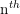harmonics of a vibrating string can be found by using the mathematical expression,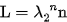Where, L - Length of the string

n - Order of harmonics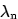- Wavelength of the nth harmonics

Rearranging this equation we get,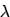---------------- (1)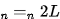We know that, wavelength of the string and frequency of the string is related by the equation,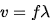-------------------- (2)

Where, v - speed of sound

f - frequency of the string

Rearranging this equation we get,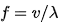? -------------------------- (3)

Step 2 of 3

Step 3 of 3

## Discover and learn what students are asking

Statistics: Informed Decisions Using Data : The Normal Approximation to the Binomial Probability Distribution
?In Problems 5–14, a discrete random variable is given. Assume the probability of the random variable will be approximated using the normal distributio

Statistics: Informed Decisions Using Data : Inference about Two Population Standard Deviations
?In Problems 9–14, assume that the populations are normally distributed. Test the given hypothesis. 10. s1 ? s2 at the a = 0.1 level of signif

Statistics: Informed Decisions Using Data : The Randomized Complete Block Design
?What is blocking? Why might a researcher want to block?

Unlock Textbook Solution